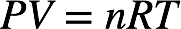🤓 Based on our data, we think this question is relevant for Professor Parnis' class at Trent University.

# Solution: A weather balloon is inflated to a volume of 28.5 L at a pressure of 748 mmHg and a temperature of 28.0 °C. The balloon rises in the atmosphere to an altitude of approximately 25,000 feet, where the pressure is 385 mmHg and the temperature is -15.0 °C. Assuming the balloon can freely expand, calculate the volume of the balloon at this altitude.

###### Problem

A weather balloon is inflated to a volume of 28.5 L at a pressure of 748 mmHg and a temperature of 28.0 °C. The balloon rises in the atmosphere to an altitude of approximately 25,000 feet, where the pressure is 385 mmHg and the temperature is -15.0 °C. Assuming the balloon can freely expand, calculate the volume of the balloon at this altitude.

###### Solution

Initially, a weather balloon has a volume of 28.5 L at 28.0 ˚C and 748 mmHg. We’re being asked to determine the volume of the weather balloon if the pressure changes to 385 mmHg and the temperature changes to –15.0 ˚C.

Recall that the ideal gas law is:The pressure, volume, and temperature of a gas are related to the number of moles of gas and the universal gas constant:The value nR is constant. For a given moles of gas, the initial and final pressure, volume, and temperature of the gas are related by the combined gas law:View Complete Written Solution